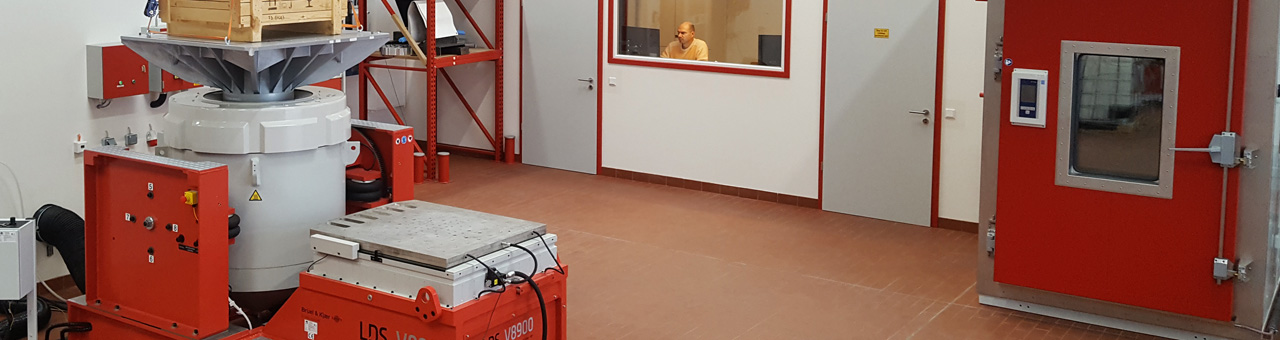# Structural Testing: Mechanical Mobility Measurements

Structural testing part two deals with mechanical mobility measurements are an important stage of the engineering design process for dynamic mechanical structures. Determination of the dynamic characteristics of structures, including noise and vibration, can be used to improve and optimize the structural performance.“STRUCTURAL TESTING PART ONE”
HANDBOOK | BRÜEL & KJÆR
RELEASE YEAR: 1988

Mechanical mobility measurements are an important stage of the engineering design process for dynamic mechanical structures. Determination of the dynamic characteristics of structures, including noise and vibration, can be used to improve and optimize the structural performance.

The main dynamic properties of a mechanical system can be defined by means of the Frequency Response Function (FRF) as the ratio of measured excitation force applied to the structure and the corresponding motion of the structure.

The motion of a structure can be described in terms of displacement, velocity or acceleration. The corresponding Frequency Response Functions are compliance, mobility and accelerance. In a general sense the term ‘mobility measurement’ is used to describe any form of FRF. For modelling, the FRF most commonly used is compliance. The FRF generally used for measurements is accelerance, since the most convenient motion transducer is the accelerometer. Compliance, mobility and accelerance are algebraically related, the measurement of any one of them can be used for calculation of the others.

## Excitation Waveform in Mobility Measurements

For mobility measurements the structure must be excited by a measurable dynamic force, but there is no theoretical restriction as to waveform, or to how the excitation is implemented.

In this discussion we will limit ourselves to waveforms which have energy distributed over a wide band of frequencies. These can simultaneously excite the structure over the entire frequency range of interest. Certain parameters should be considered before choosing the excitation waveform:

• Application
• Spectrum control
• Crest factor
• Linear/non-linear structure
• Speed of test
• Equipment available

If the purpose of the test is only to measure natural frequencies, then the precision required is much less than when the measurements are to form the basis for a mathematical model. The cost of extra precision lies in the time taken for the measurements, and in the cost of the instrumentation.

Spectrum control is the capacity to limit the excitation to the frequency range of interest. The dynamic range of an FRF is often very large, when measured between the highest resonance peak and the deepest antiresonance. Since the excitation waveform is generally chosen to have an ideally flat spectrum, it follows that the response spectrum will have the same large dynamic range as the FRF. If the structure is excited only in the frequency range of interest, the dynamic range of the measurement is minimized. This results in a better signal-to-noise ratio, and cleaner data.

The crest factor describes the ‘peakiness’ of the signal. It is defined as the ratio between the peak and the standard deviation (RMS) in the signal. A high crest factor in the excitation waveform has two disadvantages:

• The signal-to-noise ratio is decreased, since the instrumentation must make allowances for the peaks, and some of the signal is lost in the existing noise
• High peak forces may provoke non-linear behaviour in the structure

An expectation of non-linear behaviour in a structure raises the question: ‘Do we want to describe the non-linear behaviour, or do we want to make a linear approximation?

Modal analysis assumes linear systems and uses linear models. If we deal with a structure exhibiting some nonlinear behaviour, we generally attempt to make the best linear approximation. Selecting a waveform that excites the structure over a wide variety of levels randomizes the nonlinear behaviour which is then averaged out. To study nonlinearities, sinusoidal excitation with maximum amplitude control is generally used.